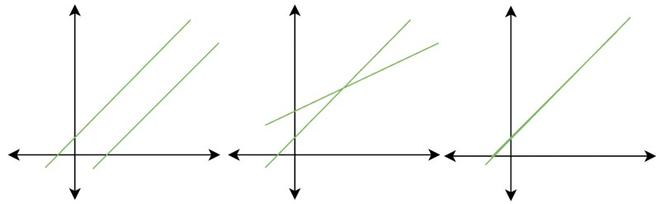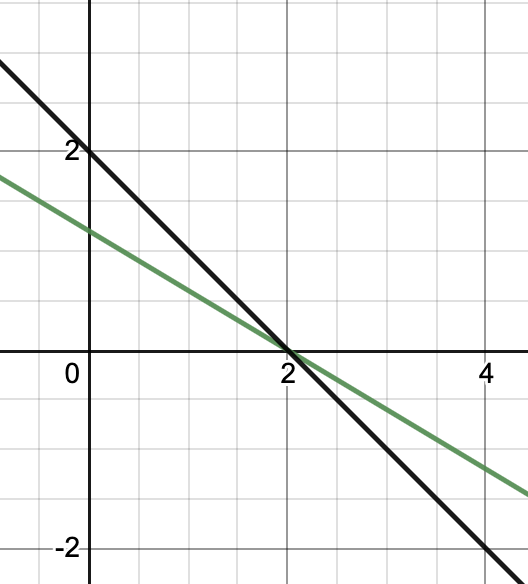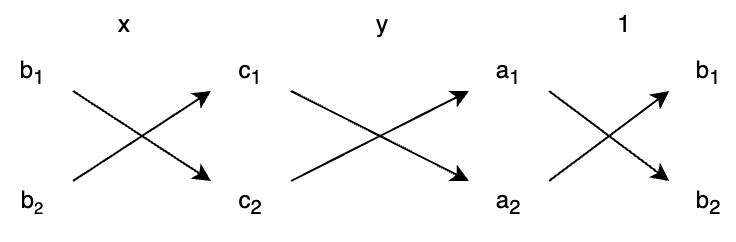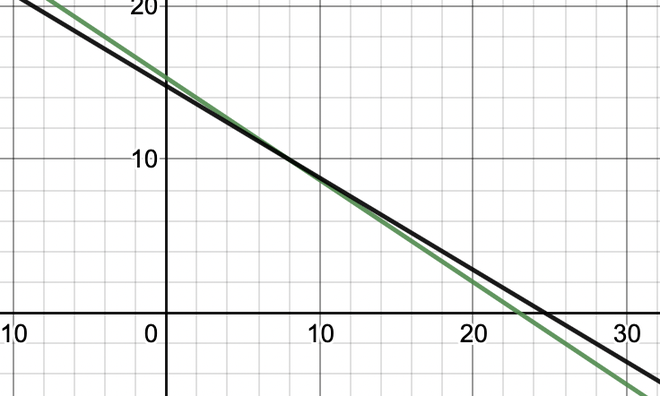# Pair of Linear Equations in Two Variables

• Last Updated : 19 Apr, 2021

Linear equations are used to describe a relationship between two variables. Sometimes in some situations, we don’t know the values of the variables we want to observe. So, then we formulate the equations describing how they behave and solve them. The Number of equations obtained should be equal to the number of variables. For example,

Camus and Bob went to a shop to buy some books. Camus bought three copies of “Metamorphosis” and four copies of “Myth of Sisyphus”. He paid Rs 500 for these books. Then, Bob bought four copies of Metamorphosis and one copy of “Myth of Sisyphus” in Rs 600. How would you find out cost of each book?

In such situations as above, making two linear equations and solving them works. There are several ways of solving such pairs of equations.

### Pair of linear equations two Variables

A linear equation is defined as,

ax + by + c = 0

Where, a, b and c are real numbers and a and b both are not zero.

A pair is formed by two such linear equations. It can be represented as,

a1x + b1y + c1 = 0

a2x + b2y + c2 = 0

a1, b1, c1, a2, b2 and c3 are real numbers.

Since a linear equation represents a line on the Cartesian plane. A pair represents two lines on the Cartesian plane. The solution to this system will be the points that satisfy both of these equations. There are three possibilities in such a system:

1. No Solution
2. Unique Solution
3. Infinitely Many SolutionsNo Solution, Unique Solution, and Infinitely Many Solutions respectively

Let’s look briefly at different methods of solving these equations.

### Graphical Methods of Solutions

In this method, we represent the equations on the graph and find out their intersection through it. We look for the points which are common to both lines, sometimes there is only a point like that, but it might also happen that there are no solutions or infinite solutions.

Question: Find out the intersection of the following lines.

3x + 5y = 6

x + y = 2

Solution:

We will plot both the lines on the graph.These lines intersect at (2,0).

If we compare the coefficients a1, a2, b1, b2, c1, and c2 with different pairs of equations which have a unique solution, infinite solutions, and no solutions. We obtain the results given below in the table.

For two lines,

a1x + b1y + c1 = 0

a2x + b2y + c2 = 0

### Algebraic Methods of Solving a Pair of Linear Equations

Substitution Method

In this method, we use one equation to express a variable in terms of the other variable thereby reducing the number of variables in the equation. Then we substitute that expression into the other equation that is given to us.

Question: Solve the following pair of equations with the substitution method.

x + y = 3

3x + y = 16

Solution:

Let’s pick the equation

x + y = 3

x = 3 – y

Substituting the value of x in the other equation,

3x + y = 16

3(3 – y) + y = 16

9 – 3y + y = 16

-2y =7

y = -7/2

Elimination Method

This method is sometimes more convenient than the substitution method. In this method, we eliminate one variable by multiplying and adding equations with suitable constants, this is done to eliminate one variable, and when the equation is left with just one variable, it can easily be solved.

Question: Solve the following equations with the elimination method.

x + y = 3

x – y = 5

Solution:

We have two equations,

x + y = 3  …….(1)

x – y = 5 …… (2)

Adding the equation (1) and (2) to eliminate the variable -y.

2x = 8

x = 4

Substituting the value of x in equation (1)

4 + y = 3

y = -1

Cross-Multiplication Method

This method looks more complex than the other methods, but it is one of the most efficient ways to solve linear equations. Let’s say the two lines whose equation is,

a1x + b1y + c1 = 0

a2x + b2y + c2 = 0

In this cross-multiplication method,The solution is given by,Question 1: Solve the following equations with the elimination method.

2x + 3y = 46

3x + 5y = 74

Solution:

a1 = 2, a2 = 3, b1 = 3, b2 = 5, c1 = 46 and c2 = 74x = 8 and y = 10

Question 2: Solve the following pair of equations graphically:

2x + 3y = 46

3x + 5y = 74

Solution:

We need to plot them of graph separately and then look at their intersection.This graph intersects are (10,8)

Question 3: Solve the following pair of linear equations with substitution method.

5x + 4y = 20

x + 2y = 4

Solution:

We have to solve these two equations

5x + 4y = 20

x + 2y = 4

Let’s we pick the second equation,

x = 4 – 2y

Now substituting the value of x in the other equation.

5(4 – 2y) + 4y = 20

20 – 10y + 4y = 20

-6y = 0

y = 0

Finding out the value of x by substituting the value of y in the equation,

x = 4 – 2y

x = 4

(4, 0) is the solution to this pair of linear equations.

Question 4: Solve the following equations with the elimination method.

4x + 5y = 20

8x + 2y = 5

Solution:

Let the equations be,

4x + 5y = 20 ……..(1)

8x + 2y = 5 ….. (2)

We need to eliminate one of the variables here from these two equations,

Multiply equation (1) with 2 and subtract it with (2).

2 x(1) -(2)

8x + 10y = 40 ….. 2 x(1)

8x + 2y = 5 …..(2)

Subtracting both of these,

8y = 35

y =Substituting this value in equation (1)My Personal Notes arrow_drop_up